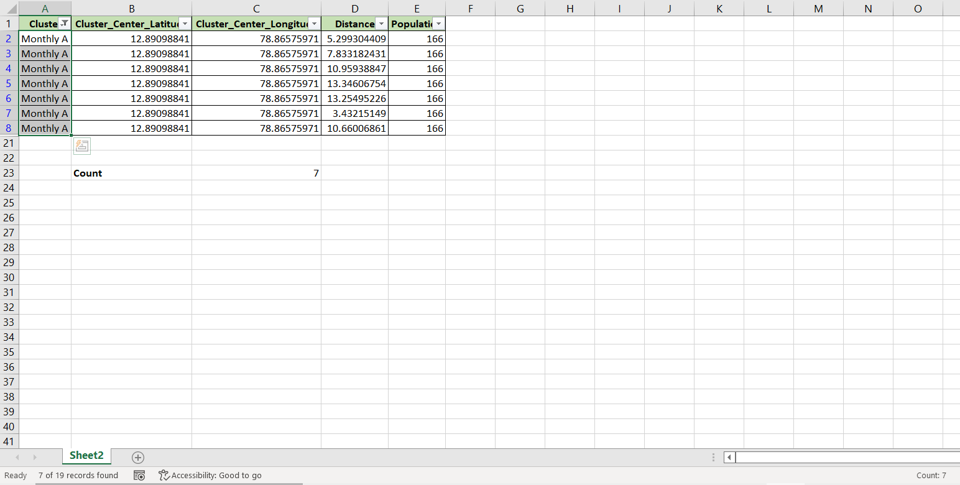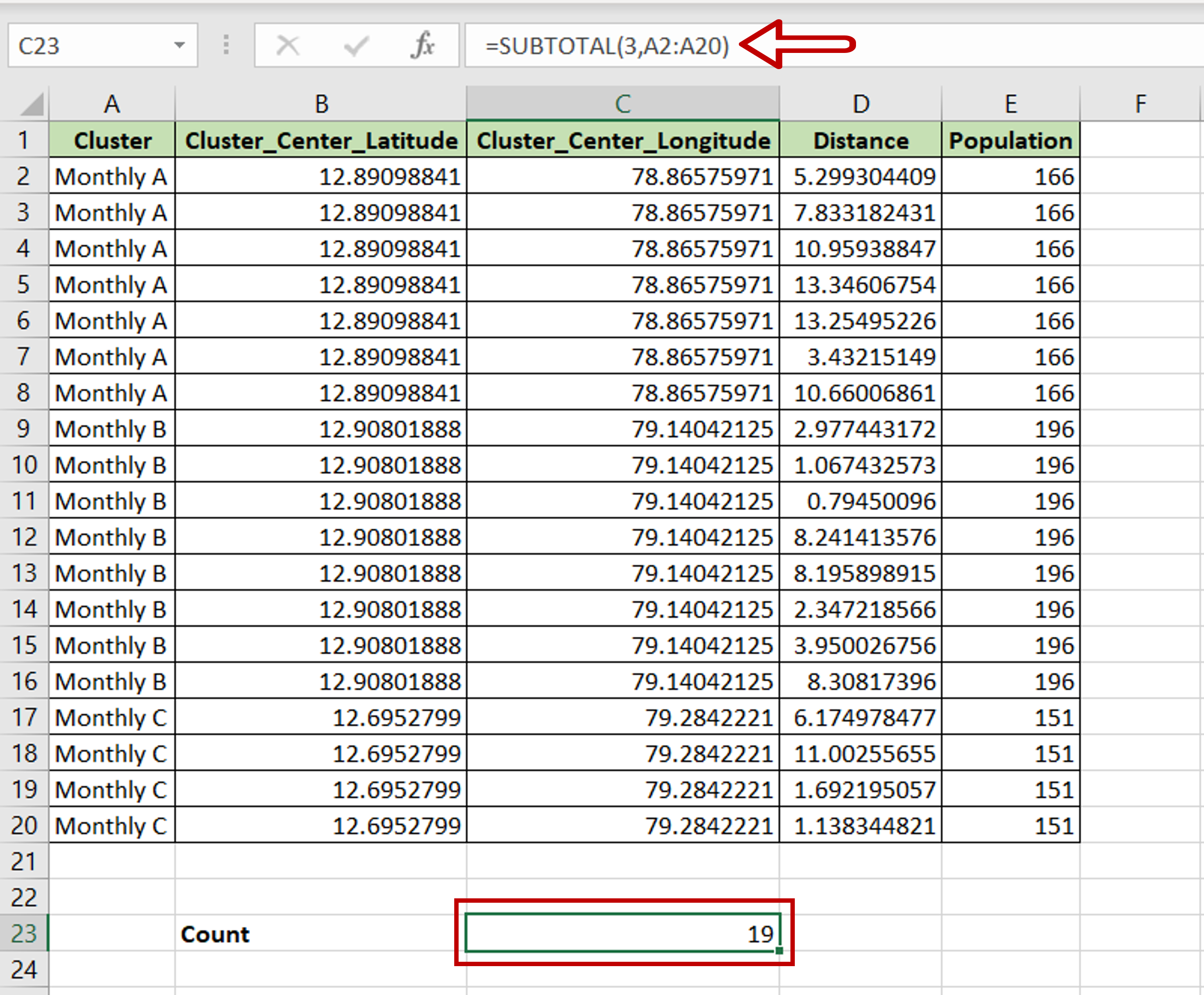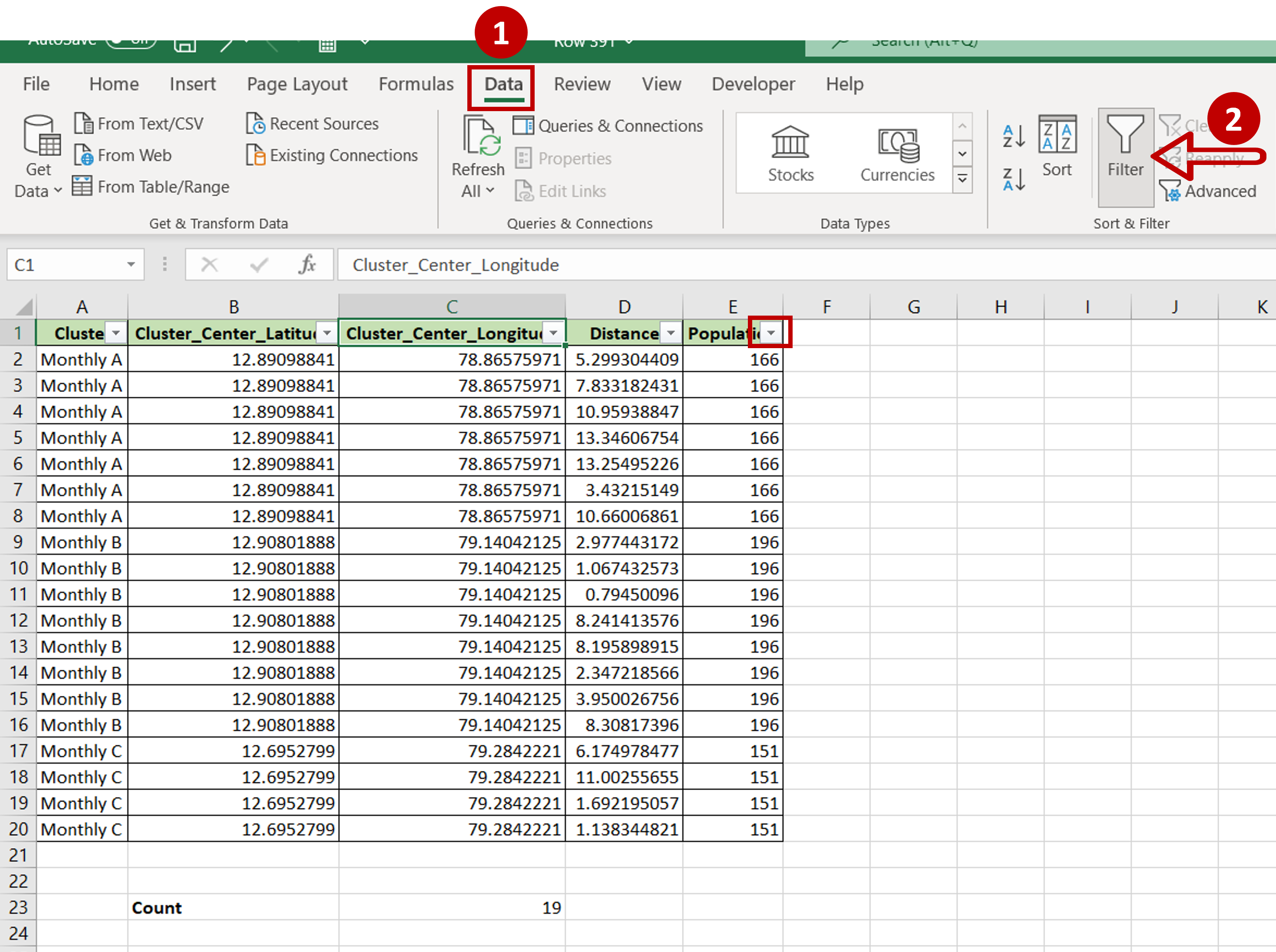# How to count filtered rows in Excel

You can watch a video tutorial here.In Excel, filters are a popular way of creating a subset of the data to analyze it or to perform data cleaning operations.  As part of data analysis, you may need to understand how many rows are returned as a result of applying a filter. You can do so using the SUBTOTAL() function and a filter.

1. SUBTOTAL() function: this returns the subtotal for a column using a specified aggregation function
a) Syntax: SUBTOTAL(function_code, ranges)
i. function_code: this is a number that corresponds to an aggregation function e.g. 1 is AVERAGE, 2 is COUNT, etc.
ii. ranges: the range of the cells on which the function is to be applied

### Step 1 – Create the Subtotal() function– Select a cell below the data to be filtered
– Type the formula using cell references:
=SUBTOTAL(3, <range of the first column>)

Note: 3 represents the COUNTA function that is used to count both numbers and text

### Step 2 – Turn on the in-column filters– Go to Data > Sort & Filter
– Click the Filter button

### Step 3 – Apply the filter– Expand the filter drop-down menu of the first column
– Click Select All to unselect all options
– Click ‘Monthly A’
– Click OK

### Step 4 – Check the count– On the bottom left of the sheet, the number of filtered cells will be displayed
– Select the filtered cells and check the bottom left of the sheet where the count of the cells will be displayed
– These numbers will match the display of the SUBTOTAL() function